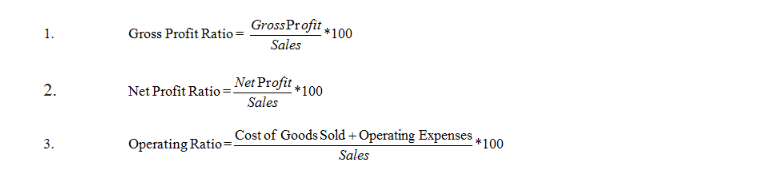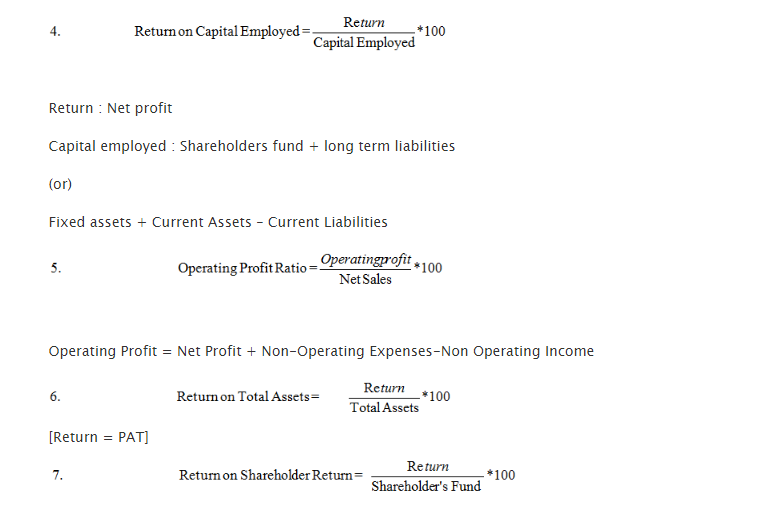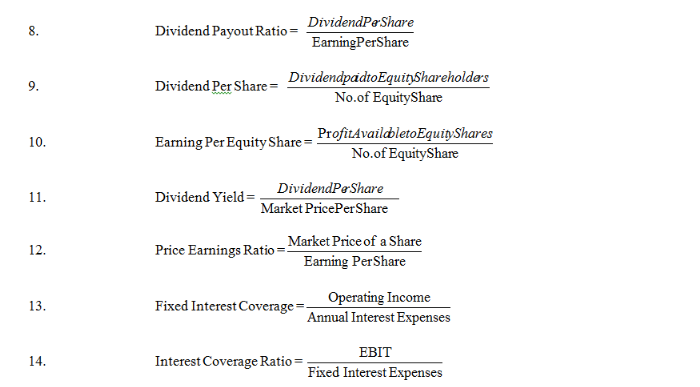Cost of Goods Sold = Sales – Gross Profit

Operating Expenses = All the expenses debited in the profit & Loss A/c

However certain expenses as financial, selling and distribution and other such minimal ones are excluded.Gross Profit Ratio = (Gross Profit/Net Sales) x 100

Gross Profit = Sales – Cost of Goods Sold

Net Sales = Gross Sales – Sales Return/Return InwardsOperating Profit Ratio =  (Operating profit x Net sales)/100

Operating Profit =  Non-Operating Expenses + Net Profit -Non Operating Income

Return on Total Assets = (Return/Total Assets) x 100

Dividend Per Share = (Dividend paid to equity shareholder’s/No of equity share)

Return on Shareholder’s Return = (Return/Shareholder’s fund) x 100

Dividend Pay-out Ratio = Dividend per share/Earning per share

Earning per Equity share = Profit available to equity shares/no of equity shares

Price earning’s ratio = Market price of share/Earning per share

Dividend Yield = Dividend per share/Market price per share

Interest Coverage Ratio = EBIT/Fixed interest expenses

Fixed Interest Coverage = Operating Income/Annual Interest Expenses### Customer Reviews

My Homework Help
Rated 5.0 out of 5 based on 510 customer reviews at